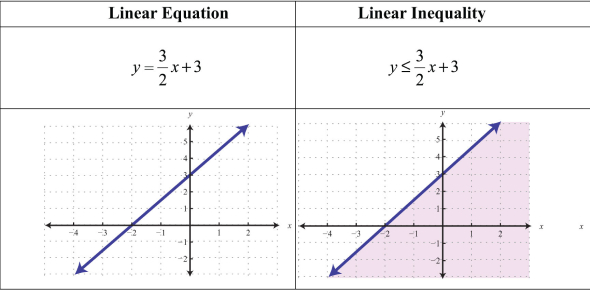# Linear Inequality Quiz

10 Questions | Attempts: 2660SettingsThis quiz was created to test student understanding at the end of a lesson on how to solve linear inequalities. The students will be required to complete the quiz online during classtime spent in the computer lab.

• 1.
To solve an inequality means to find all values of the variable for which the statement is true.
• A.

True

• B.

False

• 2.
Solve the inequality:   4x + 7 ≥ 2x – 3
• A.

X ≥ 2

• B.

X ≥ -2

• C.

X ≥ -5

• D.

X ≤ -5

• 3.
The following illustration represents the interval  [-1, 3)
• A.

True

• B.

False

• 4.
Two inequalities having exactly the same solution set are called
• 5.
Write the following inequalities using interval notation: x < -4     and     -2 ≤ x < 7
• A.

(-∞, -4) and [-2, 7)

• B.

(-4, ∞) and [-2, 7)

• C.

(-∞, -4) and (-2, 7)

• D.

[-∞, -4] and [-2, 7]

• 6.
Fill in the blank with the correct inequality symbol:  • •If x ≥ 5, then -4x ______ -20
• A.

• B.

<

• C.

>

• D.

• E.

=

• 7.
A car that averages 25 miles per gallon has a tank that holds 20 gallons of gasoline.  After a trip that covered at least 300 miles, the car ran out of gasoline.  What is the range of the amount of gasoline (in gallons) that was in the tank at the start of the trip?
• A.

15 gallons ≤ x ≤ 20 gallons

• B.

12 gallons ≤ x ≤ 20 gallons

• C.

15 gallons ≤ x ≤ 25 gallons

• D.

0 gallons ≤ x ≤ 12 gallons

• E.

12 gallons < x ≤ 20 gallons

• 8.
Solve the following linear inequality graphically:   -2x – 6 ≤ 8
• A.

X ≤ -7

• B.

X ≥ -7

• C.

X < 1

• D.

X ≥ -1

• 9.
On the graph below, f(x)= 3x - 3 (in red) and g(x)= 12  (in blue).  When is f(x) < g(x)?  Write your answer in interval notation.
• 10.
On the graph below, f(x)= -5 (in blue)  g(x)= 4-3x  (in red)  and h(x)= 2 (in green) f(x) ≤ g(x) ≤ h(x) when  2/3 ≤ x ≤ 3
• A.

True

• B.

False

## Related TopicsBack to top
×

Wait!
Here's an interesting quiz for you.